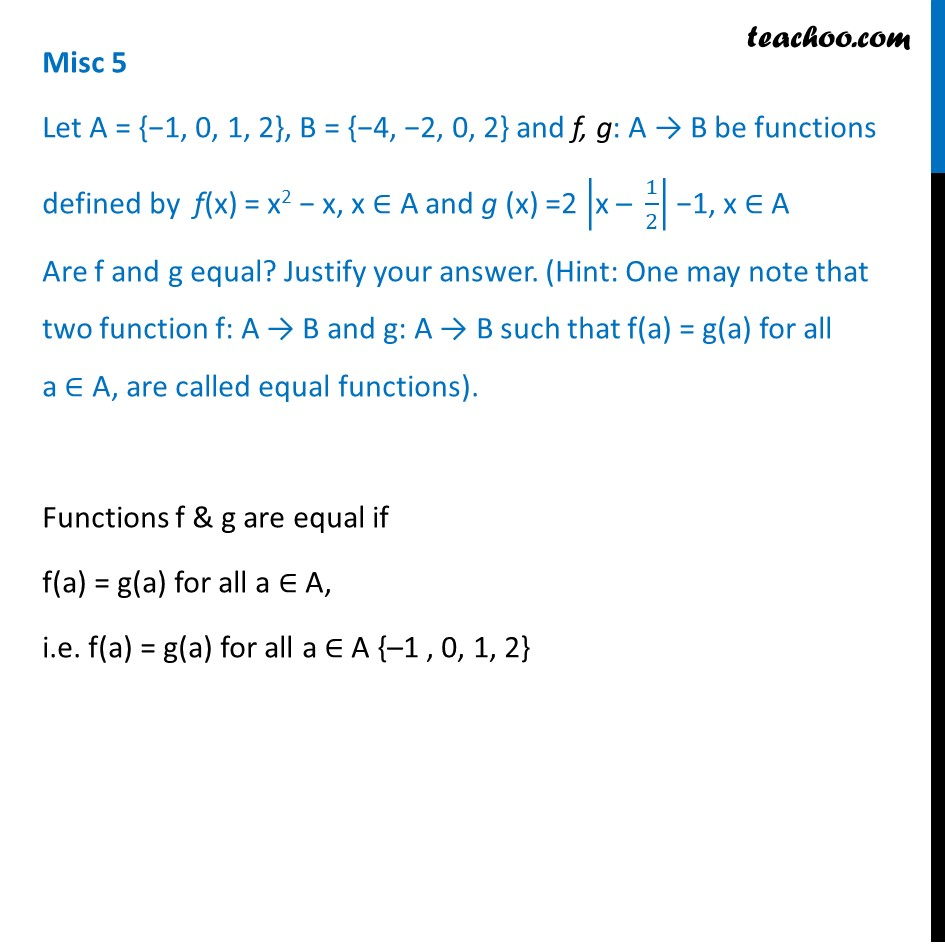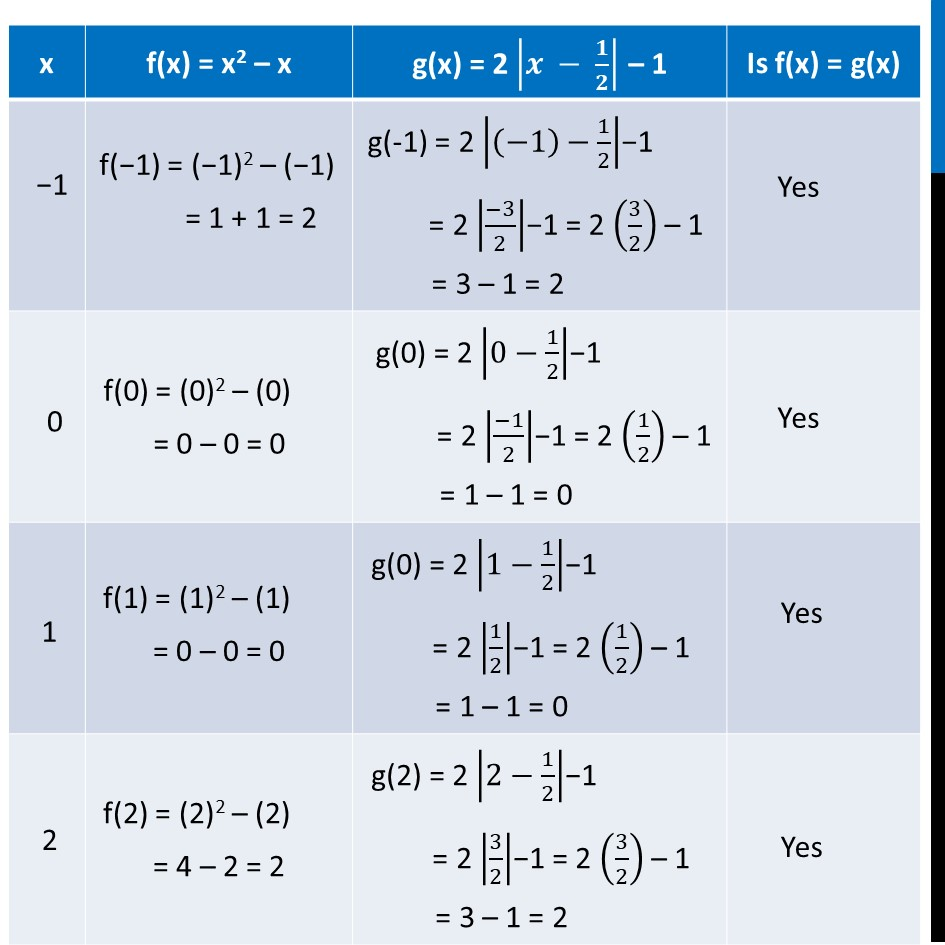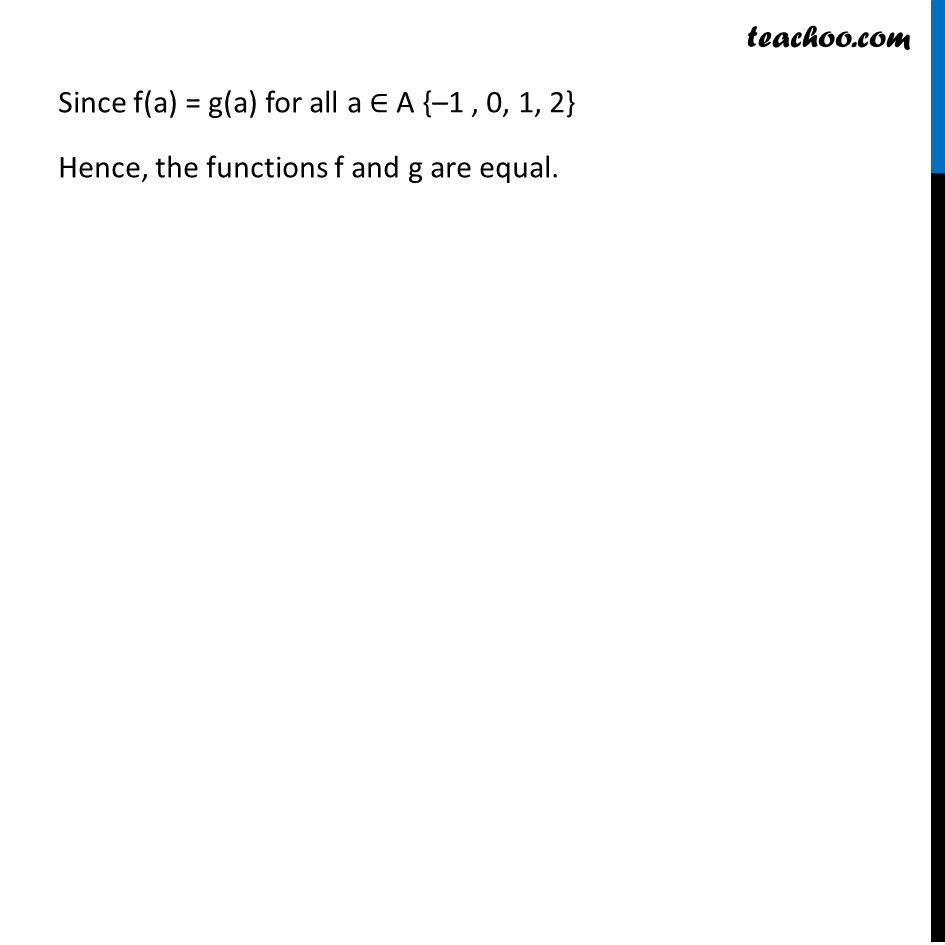Miscellaneous

Chapter 1 Class 12 Relation and Functions
Serial order wiseLearn in your speed, with individual attention - Teachoo Maths 1-on-1 Class

### Transcript

Misc 5 Let A = {−1, 0, 1, 2}, B = {−4, −2, 0, 2} and f, g: A → B be functions defined by f(x) = x2 − x, x ∈ A and g (x) =2 |"x – " 1/2| −1, x ∈ A Are f and g equal? Justify your answer. (Hint: One may note that two function f: A → B and g: A → B such that f(a) = g(a) for all a ∈ A, are called equal functions). Functions f & g are equal if f(a) = g(a) for all a ∈ A, i.e. f(a) = g(a) for all a ∈ A {–1 , 0, 1, 2} Since f(a) = g(a) for all a ∈ A {–1 , 0, 1, 2} Hence, the functions f and g are equal.